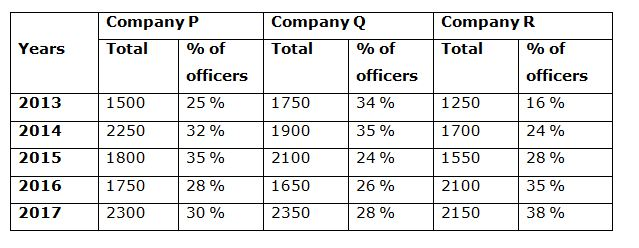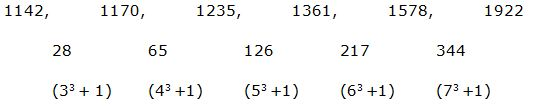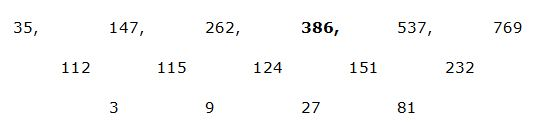# IBPS SO Prelims Quantitative Aptitude Questions 2019 (Day-5)

Dear Aspirants, Our IBPS Guide team is providing new series of Quantitative Aptitude Questions for IBPS SO Prelims 2019 so the aspirants can practice it on a daily basis. These questions are framed by our skilled experts after understanding your needs thoroughly. Aspirants can practice these new series questions daily to familiarize with the exact exam pattern and make your preparation effective.

Ensure Your Ability Before of Exam

Take IBPS SO Prelims Free Mock test

[WpProQuiz 7526]

Data interpretation

Directions (1 – 5): Study the following table carefully and answer the given questions.

Following table shows the total number of employees in 3 different companies in different years and also shows the percentage of officers among them.1) Total number of officers of company P in the year 2013 and 2015 together is approximately what percentage more/less than the total number of officers of company R in the year 2016 and 2017 together?

a) 25 % more

b) 35 % more

c) 35 % less

d) 25 % less

e) 15 % more

2) Find the respective ratio between the total number of employees of company Q to that of total number of employees of company R in all the given years together?

a) 39 : 35

b) 41 : 37

c) 23 : 19

d) 15 : 11

e) None of these

3) Find the difference between the total number of employees of other than officers in company P in the year 2014 and 2016 together to that of total number of officers of company R in the year 2013, 2014 and 2015 together?

a) 1536

b) 1924

c) 2152

d) 1748

e) None of these

4) Find the total number of employees other than officers of company Q in the year 2014, 2015 and 2016 together?

a) 3598

b) 3756

c) 4052

d) 4234

e) None of these

5) Total number of employees of company Q in the year 2014 and 2015 together is approximately what percentage of total number of employees of company R in the year 2014 and 2016 together?

a) 90 %

b) 105 %

c) 120 %

d) 135 %

e) 75 %

Wrong number series

Directions (6 – 10): Find the wrong term in the following number series?

6) 16830, 1530, 10710, 2140, 6426, 3213

a) 1530

b) 10710

c) 6426

d) 3213

e) 2140

7) 35560, 17781, 5929, 1487.5, 304.5

a) 17781

b) 1487.5

c) 5929

d) 304.5

e) 35560

8) 1142, 1170, 1235, 1360, 1578, 1922

a) 1360

b) 1170

c) 1235

d) 1578

e) 1922

9) 35, 147, 262, 385, 537, 769

a) 537

b) 385

c) 769

d) 147

e) 262

10) 81, 1000, 121, 1727, 169, 2744

a) 81

b) 121

c) 1727

d) 169

e) 2744

Directions (1-5):

Total number of officers of company P in the year 2013 and 2015 together

= > 1500*(25/100) + 1800*(35/100)

= > 375 + 630 = 1005

Total number of officers of company R in the year 2016 and 2017 together

= > 2100*(35/100) + 2150*(38/100)

= > 735 + 817 = 1552

Required % = [(1552 -1005)/1552]*100 = 35 % less

The total number of employees of company Q in all the given years together

= > 1750 + 1900 + 2100 + 1650 + 2350 = 9750

The total number of employees of company R in all the given years together

= > 1250 + 1700 + 1550 + 2100 + 2150 = 8750

Required ratio = 9750: 8750 = 39: 35

The total number of employees of other than officers in company P in the year 2014 and 2016 together

= > 2250*(68/100) + 1750*(72/100)

= > 1530 + 1260 = 2790

The total number of officers of company R in the year 2013, 2014 and 2015 together

= > 1250*(16/100) + 1700*(24/100) + 1550*(28/100)

= > 200 + 408 + 434 = 1042

Required difference = 2790 – 1042 = 1748

The total number of employees other than officers of company Q in the year 2014, 2015 and 2016 together

= > 1900*(65/100) + 2100*(76/100) + 1650*(74/100)

= > 1235 + 1596 + 1221 = 4052

Total number of employees of company Q in the year 2014 and 2015 together

= > 1900 + 2100 = 4000

Total number of employees of company R in the year 2014 and 2016 together

= > 1700 + 2100 = 3800

Required % = (4000/3800)*100 = 105 %

Directions (6-10):

The correct series is,

16830, 1530, 10710, 2142, 6426, 3213

The pattern is, ÷ 11, *7, ÷ 5, *3, ÷ 2,..(Prime no’s)

The wrong term is, 2140

The correct series is,

35560, 17781, 5930, 1487.5, 304.5

The pattern is, ÷ 2 +1, ÷ 3 +3, ÷ 4 +5, ÷ 5 +7,..

The wrong term is, 5929

The correct series is,

1142, 1170, 1235, 1361, 1578, 1922The difference is, 33 +1, 43 +1, 53 +1, 63 +1, 73 +1

The wrong term is, 1360

The correct series is,The difference of difference is, *3

The wrong term is, 385

The correct series is,

81, 1000, 121, 1728, 169, 2744

The pattern is, 92, 103, 112, 123, 132, 143,…

The wrong term is, 1727